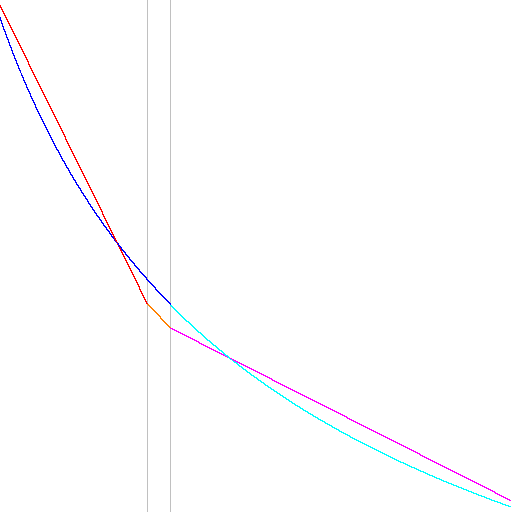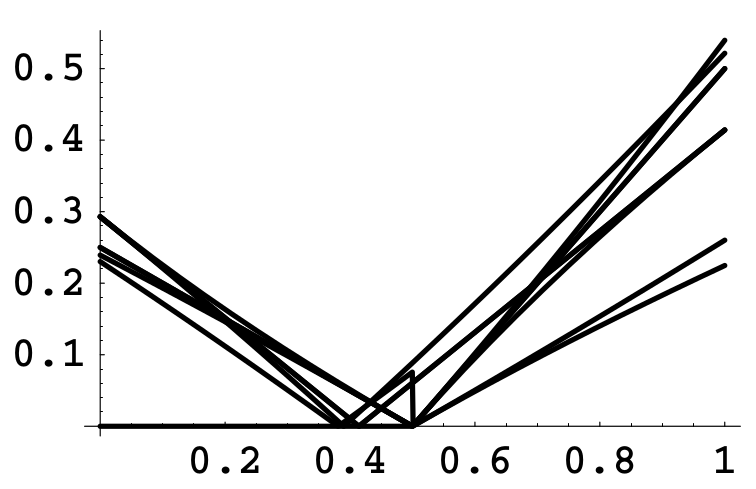Fast Inverse Square Root

This is a classic hack in the graphics community. It is not optimal on modern hardware, but is still useful for some smaller systems. This hack has been in use since the 70s.

Here's an example from the Quake3 source code:

float Q_rsqrt( float number )
{
long i;
float x2, y;
const float threehalfs = 1.5F;

x2 = number * 0.5F;
y  = number;
i  = * ( long * ) &y;                       // evil floating point bit level hacking
i  = 0x5f3759df - ( i >> 1 );               // what the fuck?
y  = * ( float * ) &i;
y  = y * ( threehalfs - ( x2 * y * y ) );   // 1st iteration
//  y  = y * ( threehalfs - ( x2 * y * y ) );   // 2nd iteration, this can be removed

return y;
}

In order to understand this hack, three things need to be reviewed:

1. The effect of basic operations on numbers and binary patterns
2. The structure of the floating point binary format
3. Newton's method for finding roots

Basic operations

Exponent multiplication works like this: $$2^x \times 2^y = 2^{xy}$$

Shifts in binary work as the do in decimal. In decimal: $$23 \times 10 = 230$$ $$23 << 1 = 230$$

In binary: $$0101_2 \times 2 = 1010_2$$ $$0101_2 << 1 = 1010_2$$

Floating point format

We will only consider single precision IEEE-754 floating point for this problem. Floats are 32 bits long and have this form:

1    8               23
|-|--------|-----------------------|
s    E               M

Where $$s$$ is the sign, with 0 for positive and 1 for negative. $$E$$ is the exponent, with a bias of 127. Sometimes the exponent is written as $$e$$ without the bias, so $$e = E-127$$. $$M$$ is the mantissa, with an implied leading 1. The mantissa can have a leading 0 in some special cases, which we will ignore. So the basic formula for computing a float value is: $$f = -1^s \times 1.M \times 2^{E-127}$$

A simple version

A less optimized and simpler version of the trick is:

float inverseSqrt( float number )
{
float est;
const float one = 1.0f;
int i = *(int*)&one;
i = i + (i >> 1);
i = i - *(int*)&number;
est = *(float*)&i;
return est;
}

This is based on Blinn's write up (see references). This one works by dividing the exponent by 2. A bitwise right shift should normally do this, but since the exponent field is biassed, it takes more.

The number 1 has a exponent of 0 and a mantissa of 0. So, the biassed exponent for 1 is 127. By adding the 1's exponent to its right shifted value, it approximates an E value of 190 (with some error in the mantissa): $$E = 127 + (127>>1)$$

The input value has an exponent bias of 127 and while shifting it divides the exponent by two (resulting in the square root), it leaves a bias of 63. So, subtracting the shifted input exponent removes the extra 63 from the shifted one exponent, leaving a bias of 127 and the negative shifted input exponent.

There is now some error data in the mantissa from the shift, but the exponent should be the original exponent negated and divided by two: the inverse square root with a bit of error.

Newton's method

Newton's method is an algorithm for finding roots (solutions to equations). Newton's method is: $$x_1 = x_0 - \frac{f(x_0)}{f'(x_0)}$$

That is, given an estimated solution to a function $$f$$ ($$x_0$$), a better solution can be found by following the slope of the tangent (derivative) back towards the x-axis.

Using Newton's method

So, given the function we wish to solve is $$\frac{1}{\sqrt{x}}$$, we will need to estimate a value, then use Newton's method to improve the value. However, instead of using $$\frac{1}{\sqrt{x}}$$, we will instead use Newton's method on a function that represents the error of our guess and $$\frac{1}{\sqrt{x}}$$. That is, when this error function reaches 0, we have the exact solution to the inverse square root. So, we will use Newton's method to find values that minimize the result.

Since the inverse square root is: $$\frac{1}{\sqrt{x}}$$ Given an initial value of $$x$$ and a very good guess $$y$$, then this could be true: $$y = \frac{1}{\sqrt{x}}$$ However, since $$y$$ is an estimate it will more likely be: $$y \approx \frac{1}{\sqrt{x}}$$

Given this, we wish to minimize the error between the initial value $$x$$ and the guess $$y$$. The relation between our guess is: $$\frac{1}{y^2} = x$$

The error between the guess and the actual value is then: $$\epsilon = \frac{1}{y^2} - x$$ or $$\epsilon = y^{-2} - x$$

If the error was 0, then $$y$$ would be the exact answer. Using Newton's method, the error can be minimized: $$y_1 = y - \frac{\epsilon(y)}{\epsilon'(y)}$$

The derivative of the error is: $$\epsilon'(y) = -2y^{-3}$$

Newton's method becomes:

$$y_1 = y - \frac{y^{-2} - x}{-2y^{-3}} \$$ $$y_1 = y - (y^{-2} - x)(-\frac{1}{2}y^{3}) \$$ $$y_1 = y - (-\frac{1}{2})(y - xy^{3}) \$$ $$y_1 = y - (-\frac{1}{2}y + \frac{1}{2}xy^{3}) \$$ $$y_1 = y + \frac{1}{2}y - \frac{1}{2}xy^{3} \$$ $$y_1 = \frac{3}{2}y - \frac{1}{2}xy^{3} \$$ $$y_1 = 1.5y - 0.5xy^{3} \$$ $$y_1 = y(1.5 - 0.5xy^{2}) \$$

This is the last lines of the code:

y  = y * ( threehalfs - ( x2 * y * y ) );

The exponent

Since the value of the float is $$f = -1^s \times 1.M \times 2^{E-127}$$

and we are trying to approximate $$y = \frac{1}{\sqrt{x}}$$

we can assume that $$x$$ is positive and the estimate becomes: $$y = \frac{1}{\sqrt{x}} = \frac{1}{\sqrt{1.M}} \times 2^\frac{1}{\sqrt{e}}$$ or $$y = \frac{1}{\sqrt{1.M}} \times 2^{\frac{-e}{2}}$$

The exponent has a bias of 127, so the actual exponent value is $$E = -e/2 + 127$$

We wish to divide the original exponent by 2 and negate it, but still retain the bias. This can be done in integer math as:

newE = (0 - ((E-127) >> 1)) + 127

newE = 0 - ((E >> 1)-(127 >> 1)) + 127

newE = 0 - (E >> 1) + 63 + 127

newE = 190 - (E >> 1)

or

0xbe - (E >> 1)

This works because E already has a bias of 127, so shifting by 2 divides the bias to 63. Then, subtracting the 63 results in a final bias of 127. The $$e$$ part of the exponent is thus shifted (divided by 2) and still biased correctly.

The mantissa

Since there is a bitwise shift, some bits of the exponent might end up in the mantissa. There are two cases to consider with the exponent $$E$$ of the input value: it can be odd or even. If the $$E$$ is even, no new bits will be shifted into the mantissa. This is the only case we will consider. The odd case is similar, but longer and more complex, so see the references.

If $$E$$ is even, the $$e$$ must be odd because: $$e = E - 127$$

$$e$$ can then be written as $$2d+1$$. The guess float values can be written as $$E_g$$ and $$M_g$$. Then, since the estimate will subtract the shifted initial value: $$y = (1+M_g - M/2)2^{E_g - 190 - d}$$

Since the original exponent will be shifted and has a bias of 127, it will retain a bias of 63. If the guess float values are written as $$E_g$$ and $$M_g$$, the estimate can be written as: $$y = (1+M_g - M/2)2^{E_g - 190 - d}$$ Moving a two from the exponent to the mantissa: $$y = (2+2M_g - M)^{E_g - 191 - d}$$

If $$M/2 > M_g$$, then the mantissa will borrow from the exponent, increasing the mantissa by one and dividing the exponent by two, resulting in: $$y = (1 + (1+M_g - M/2))2^{E_g - 190 - d -1}$$ $$y = (2+M_g - M/2)2^{E_g - 191 - d}$$

There are then two possible results for the mantissa estimate: $$2M_g - M \: \text{:} \: E is even, M_g \geq M/2\$$ $$M_g - M/2 \: \text{:} \: E is even, M_g < M/2$$

Similarly, there are two results for the odd case: $$4M_g - M \: \text{:} \: E is odd, M_g \geq M/2\$$ $$1 + 2M_g - M \: \text{:} \: E is odd, M_g < M/2$$

These functions represent estimates for the inverse square root. Given appropriate guesses for $$M_g$$ and $$E_g$$, they form a piecewise linear function that approximates the inverse square root curve. For the classic constant value, one of the cases can never occur, resulting in a three-part function.

Eberly plots it as:While the classic exponent can be derived, no one knows how the classic mantissa was derived. Lomont approximates it by minimizing the maximum error along the piecewise function. Minimizing this error over the function means optimizing nine possible cases.

Lomont shows the $$M_g$$ error as:Note that the error is smallest near 0.45. If the E part of 0x5f3759df is set to 127, the result will be $$1.M$$. The constant 0x3fb759df does this, which shows the mantissa have a value of 0.432430.

Better guesses for a constant have been computed and they are close to the classic estimate. The actual source or method that generated the classic constant is not known.

Example code

Here's a small program for testing the method. It will also time various inverse square root methods. On modern PCs, the standard floating point calculation is probably faster. The number of Newton iterations greatly impacts performance of the estimate calculations, so experiment with those and decide if the accuracy is worth the cost in the integer hacks.